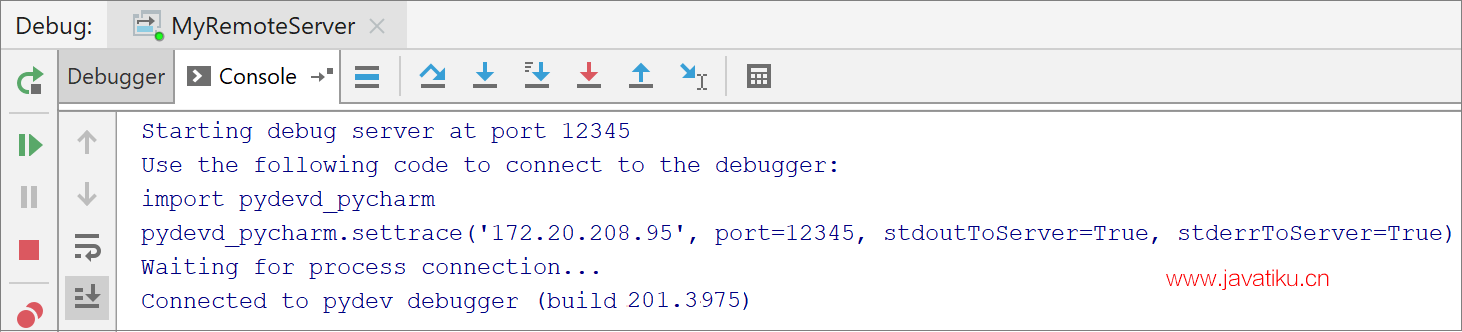# 【PyCharm教程】PyCharm 使用 PyCharm 进行远程debugger调试PyCharm 提供了两种远程调试方式：

• 通过远程翻译。

• 使用 Python远程调试服务器配置。

## 在你开始之前﻿

### 完成以下准备任务：﻿

1. 在本地机器上，创建一个纯 Python 项目，如创建 Python 项目一节中所述。
2. 将 Python 文件添加到此项目 ( Alt+Insert- Python File )。
3. 将以下代码添加到Python 文件中：

``````import math

class Solver:
def demo(self, a, b, c):
d = b ** 2 - 4 * a * c
if d > 0:
disc = math.sqrt(d)
root1 = (-b + disc) / (2 * a)
root2 = (-b - disc) / (2 * a)
return root1, root2
elif d == 0:
return -b / (2 * a)
else:
return "This equation has no roots"

if __name__ == '__main__':
solver = Solver()

while True:
a = int(input("a: "))
b = int(input("b: "))
c = int(input("c: "))
result = solver.demo(a, b, c)
print(result)``````

## 为远程解释器创建部署配置﻿

### 配置远程解释器﻿

1. 确保您对远程计算机具有 SSH 访问权限。
2. 将新的远程解释器添加到项目中，如使用 SSH 配置解释器中所述，指定连接到远程计算机的凭据。
3. 为项目创建远程解释器后，就会创建相应的部署配置。要预览它，请单击Ctrl+Alt+S以在本地计算机上打开“设置”对话框窗口，然后单击“构建”、“执行”、“部署”节点和“部署”节点。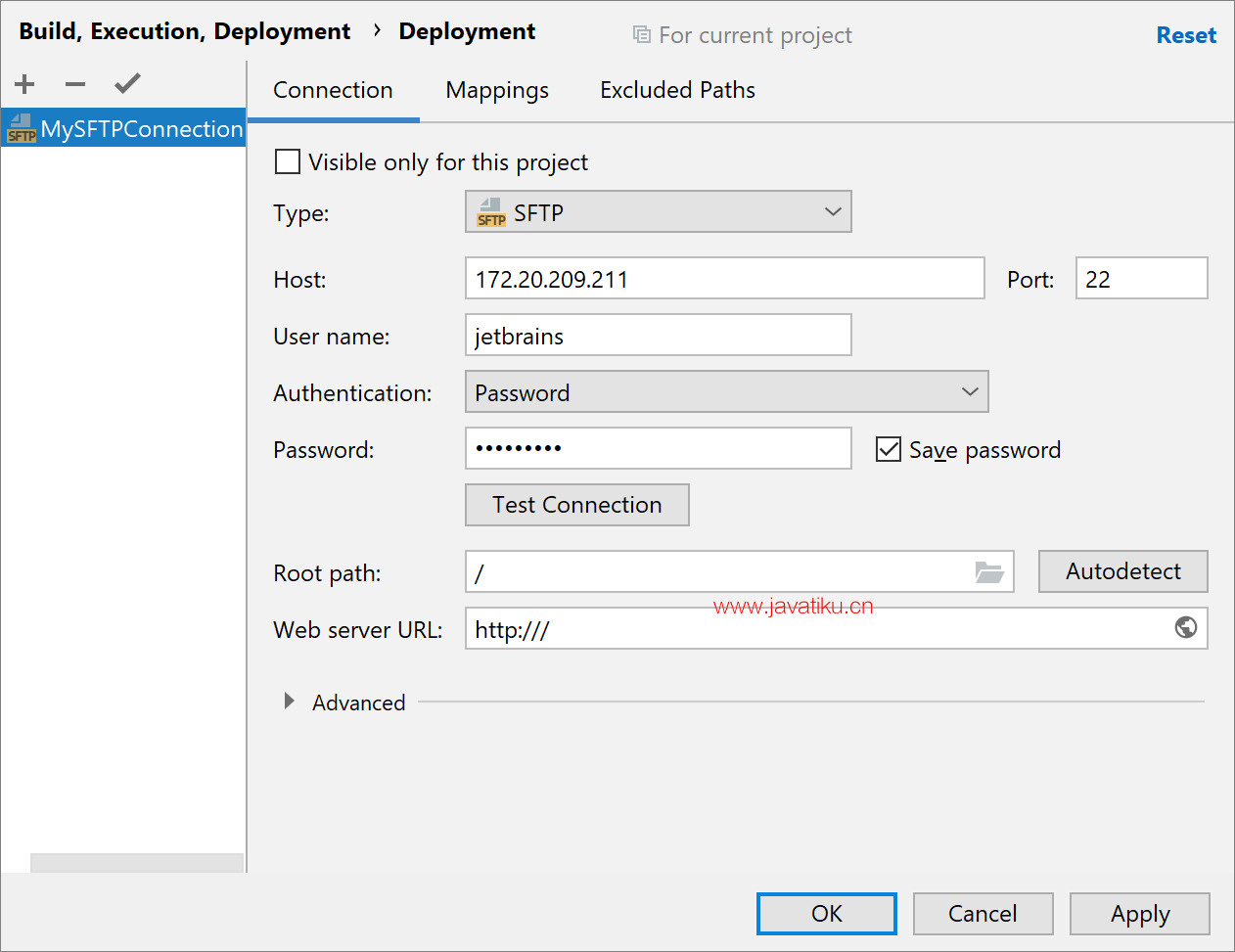1. 如果需要，您可以接受所有默认设置或更改它们。对于此示例，让我们为您的部署配置使用一个有意义的名称，例如“MySFTPConnection”。
2. 确保Root 路径值反映了在创建的 SSH 解释器的相应设置中指定的路径。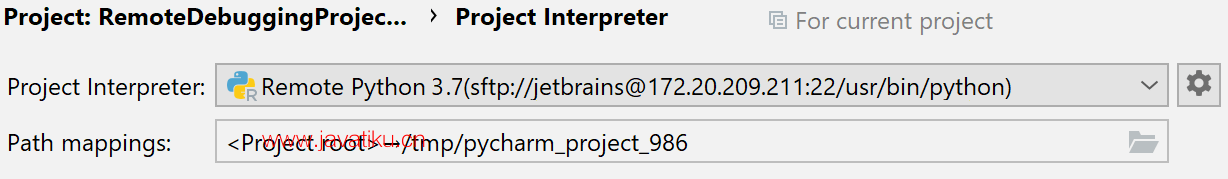### 将您的应用程序部署到远程主机﻿

1. 在工具菜单上，选择部署 | 上传到 MySFTPConnection。
2. 出现文件传输工具窗口。验证传输文件的数量。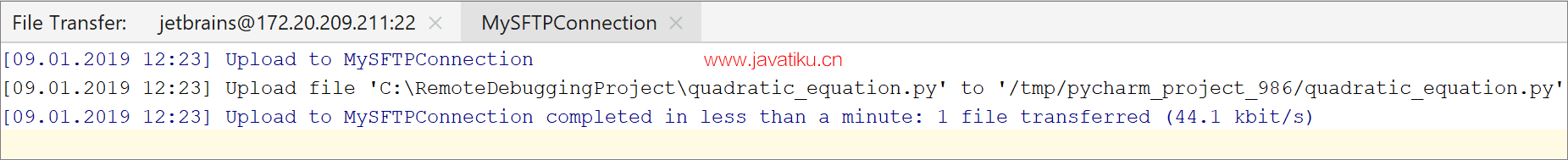### 调试您的应用程序﻿

2. 查看调试输出。请注意，调试实际上发生在指定的远程服务器上。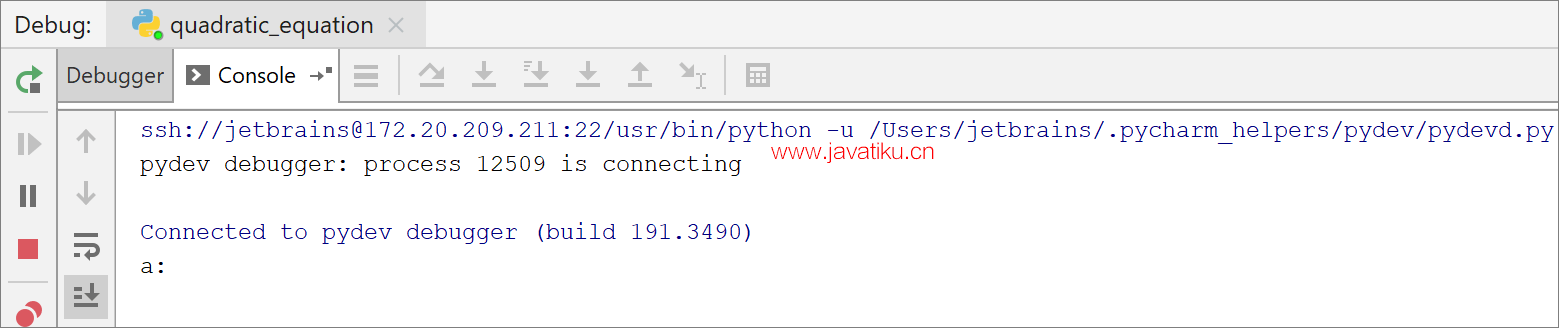## 使用 Python 远程调试服务器配置进行远程调试﻿

### 创建运行/调试配置﻿

1. 从主菜单中，选择运行| 编辑配置... . 运行/调试配置对话框打开。您必须单击工具栏上的 ，然后从可用配置列表中选择Python Debug Server。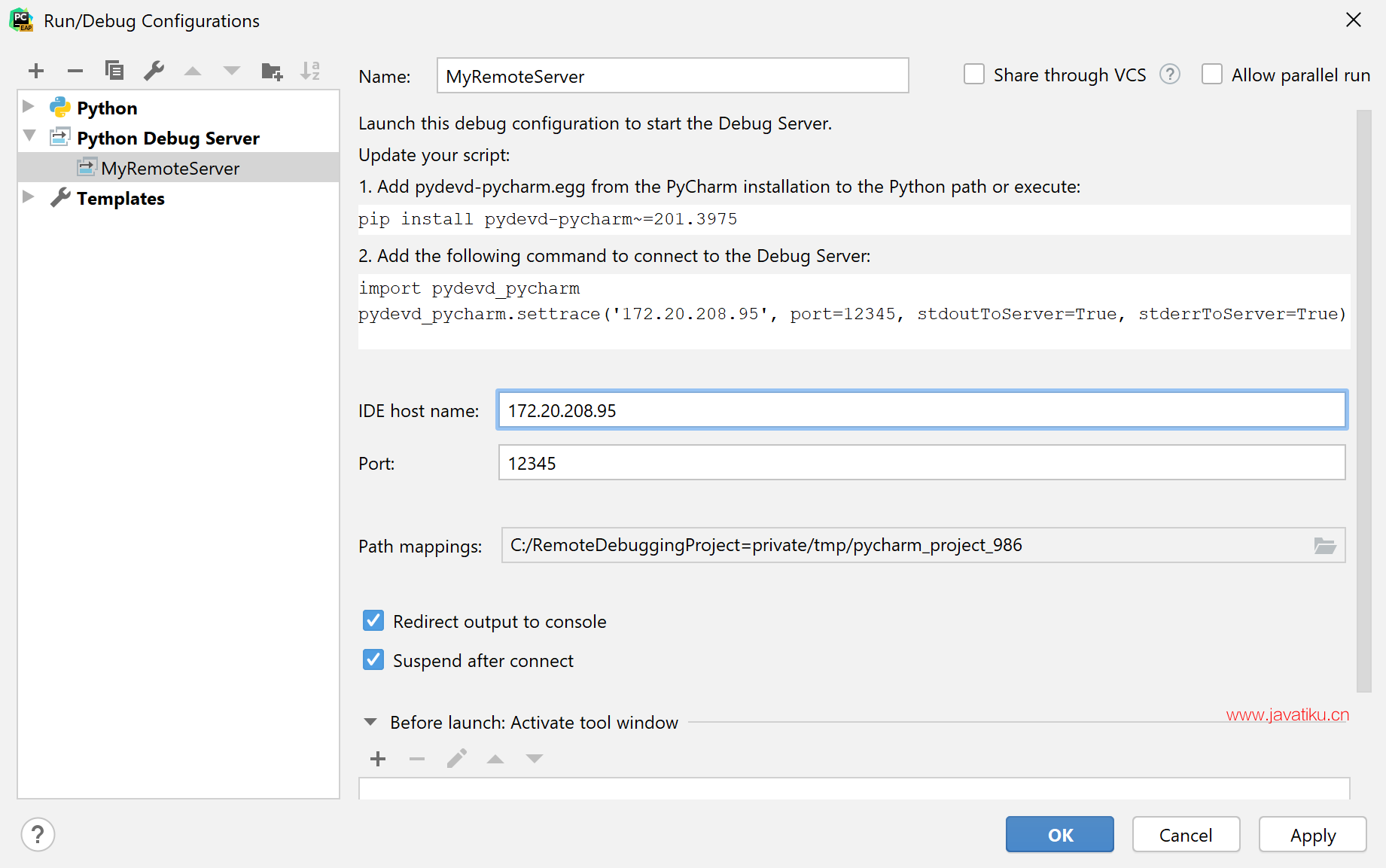1. 输入此运行/调试配置的名称 - 让它成为MyRemoteServer。指定运行 IDE 的机器的端口号（此处为12345）和IDE 主机名（此处为 172.20.208.95）。远程调试服务器将使用这些参数来访问它。
2. 将本地机器上的路径映射到远程机器上的路径：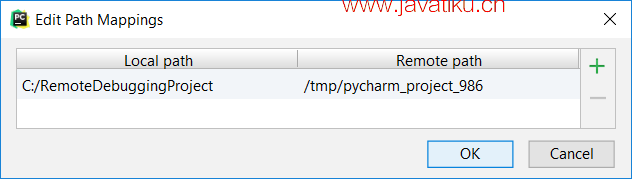1. 检查更新您的脚本说明。您可以使用PyCharm 安装（<PyCharm directory> /debug-egg /pydevd-pycharm.egg ）中的pydevd -pycharm.egg 或使用 pip安装包。`pydevd-pycharm`

• `pydevd-pycharm`通过运行以下命令在远程计算机上安装软件包：

`````` `pip install pydevd-pycharm~=<version of PyCharm on the local machine>`

例如，`pip install pydevd-pycharm~=191.3490)`
``````
• 修改源代码文件如下：

1. math
2. pydevd_pycharm

pydevd_pycharm.settrace('172.20.208.95', port=12345, stdoutToServer=True,

``                stderrToServer=True)``

#================================================================================================

3. Solver:

def demo(self, a, b, c):

``````d = b ** 2 - 4 * a * c
if d > 0:
disc = math.sqrt(d)
root1 = (-b + disc) / (2 * a)
root2 = (-b - disc) / (2 * a)
return root1, root2
elif d == 0:
return -b / (2 * a)
else:
return "This equation has no roots"
``````
4. name == '__main__':
solver = Solver()
5. True:
a = int(input("a: "))
b = int(input("b: "))
c = int(input("c: "))
result = solver.demo(a, b, c)
print(result)

``````import math
import sys
sys.path.append("<PyCharm directory>/debug-egg/pydevd-pycharm.egg")
import pydevd_pycharm

pydevd_pycharm.settrace('172.20.208.95', port=12345, stdoutToServer=True,
stderrToServer=True)
#================================================================================================
class Solver:

def demo(self, a, b, c):
d = b ** 2 - 4 * a * c
if d > 0:
disc = math.sqrt(d)
root1 = (-b + disc) / (2 * a)
root2 = (-b - disc) / (2 * a)
return root1, root2
elif d == 0:
return -b / (2 * a)
else:
return "This equation has no roots"

if __name__ == '__main__':
solver = Solver()

while True:
a = int(input("a: "))
b = int(input("b: "))
c = int(input("c: "))
result = solver.demo(a, b, c)
print(result)``````

### 创建 SFTP 连接﻿

``````\$cd /tmp
\$mkdir pycharm_project_986``````
2. 在本地计算机上，创建一个连接配置文件。从主菜单中，选择工具 | 部署 - 配置...... 在打开的对话框中，单击，然后在Add Server对话框中选择连接类型（此处为 SFTP）并输入其名称（此处为 MySFTPConnection）。
3. 在“连接”选项卡中，指定 SFTP 主机（远程机器的地址）、该机器的用户名和密码。

1. 单击映射选项卡，然后在服务器中输入部署路径。服务器是`MySFTPConnection`，所以单击浏览按钮并选择所需的文件夹/tmp /pycharm_project_986。请注意，浏览按钮显示远程主机的内容。应用更改并关闭对话框。

### 将文件部署到远程机器﻿

1. 检查“文件传输”对话框窗口以确保将本地计算机中的文件上传到远程服务器。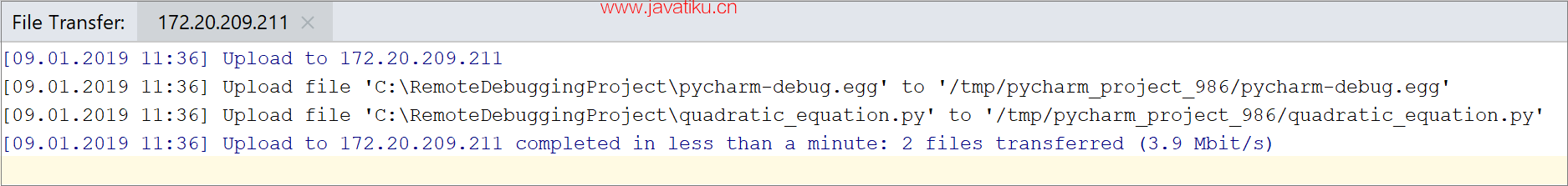### 启动调试服务器﻿

1. 选择创建的运行/调试配置，然后单击1. 确保Debug 窗口显示Waiting for process connection..消息。此消息将一直显示，直到您在远程计算机上启动脚本，并且此脚本将连接到调试服务器。

### 在远程机器上执行 Python 文件﻿

1. 在远程机器上，导航到tmp/pycharm_project_986目录。

python 3

`\$python3 quadratic_equation.py`

python 2

`\$python quadratic_equation.py`

python 3

`cd /tmp/pycharm_project_986`

`python3 quadratic_equation.py`

python 2

`cd /tmp/pycharm_project_986`

`python quadratic_equation.py`

### 调试您的应用程序﻿

• 在本地机器上，切换到Debug 窗口。它应该显示与 pydev 调试器的连接。